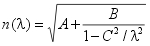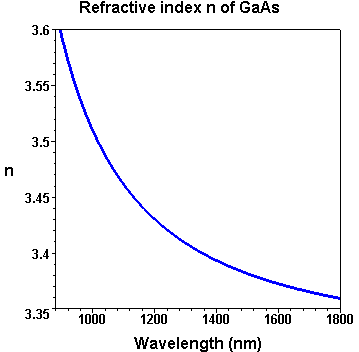> Energy band gap> GaAs | AlxGa1-xAs | InxGa1-xAs
> Refractive index > GaAs | AlAs | AlxGa1-xAs | InxGa1-xAs
> Devices > Bragg mirror | SAM | RSAM | SA | SANOS | SOC | Microchip | PCA
> Device application > Papers | Patents | FAQs## Refractive index n of GaAs

### Sellmeyer equation

In the energy range below or near the fundamental absorption edge the dispersion of the refractive index n(λ) of GaAs can be calculated by the first-order Sellmeier equation:In this equation the symbols and constants have the following meaning in the case of GaAs at room temperature:

• λ - vacuum wavelength in µm
• A = 8.950 - empirical coefficient
• B = 2.054 - empirical coefficient
• C2 = 0.390 - empirical coefficient

### Numerical values

λ (nm) n(GaAs)
850 3.655
900 3.593
950 3.545
1000 3.510
1050 3.483
1100 3.461
1200 3.430
1300 3.408
1400 3.393
1500 3.382
1700 3.365
2000 3.351